# Binomial Theorem - Exercise 2.1 : Class 12 Math

Binomial Theorem - Exercise 2.1 : Class 12 Mathematics. Complete Binomial Theorem Exercise 2.1 of Class 12 Math, NEB 2080.

## Chapter 2: Binomial Theorem.

### Exercise: 2.1

Complete Exercise of Binomial Theorem - Exercise 2.1 : Class 12 Mathematics 2080 NEB.

Read: NEB Class 12 Mathematics All Chapter Exercise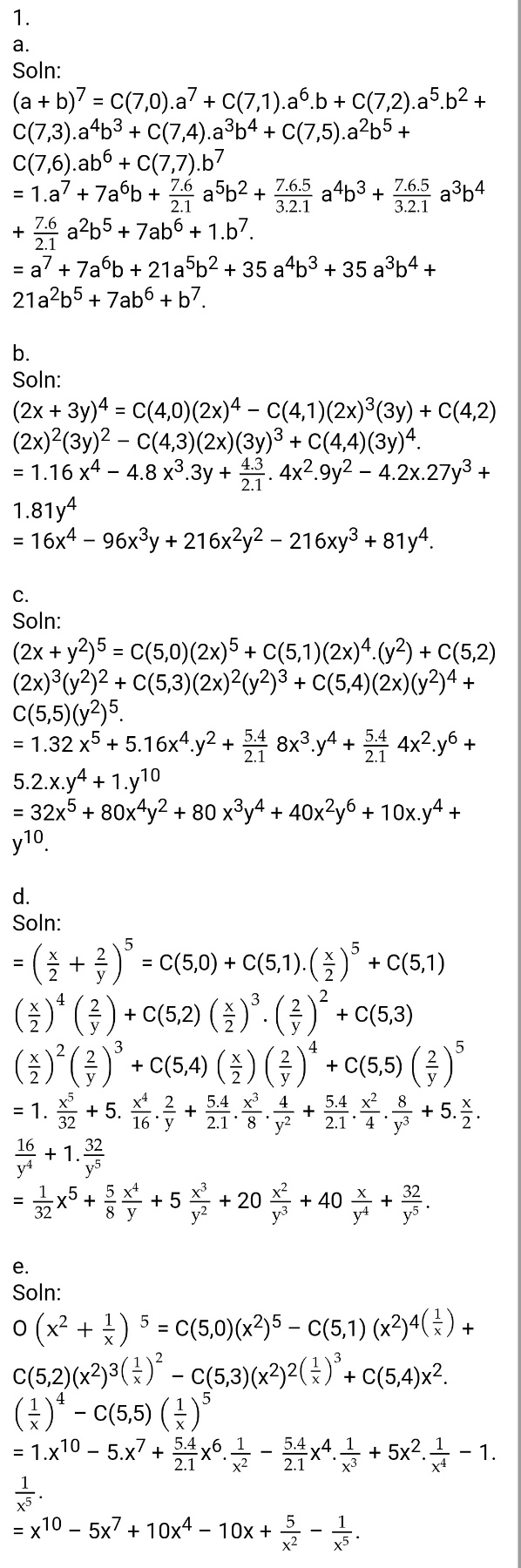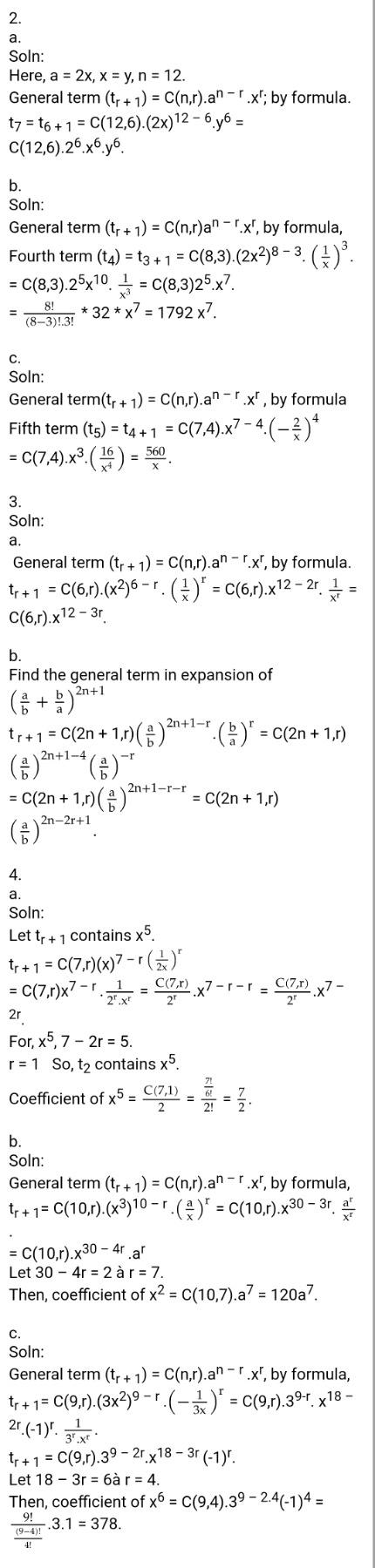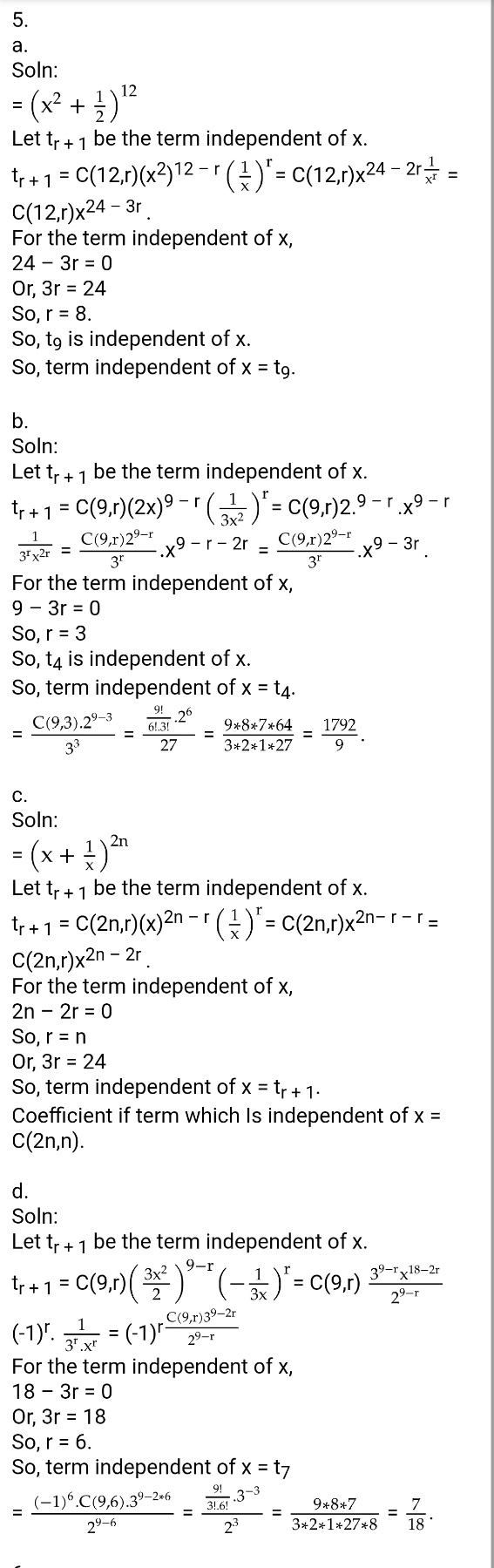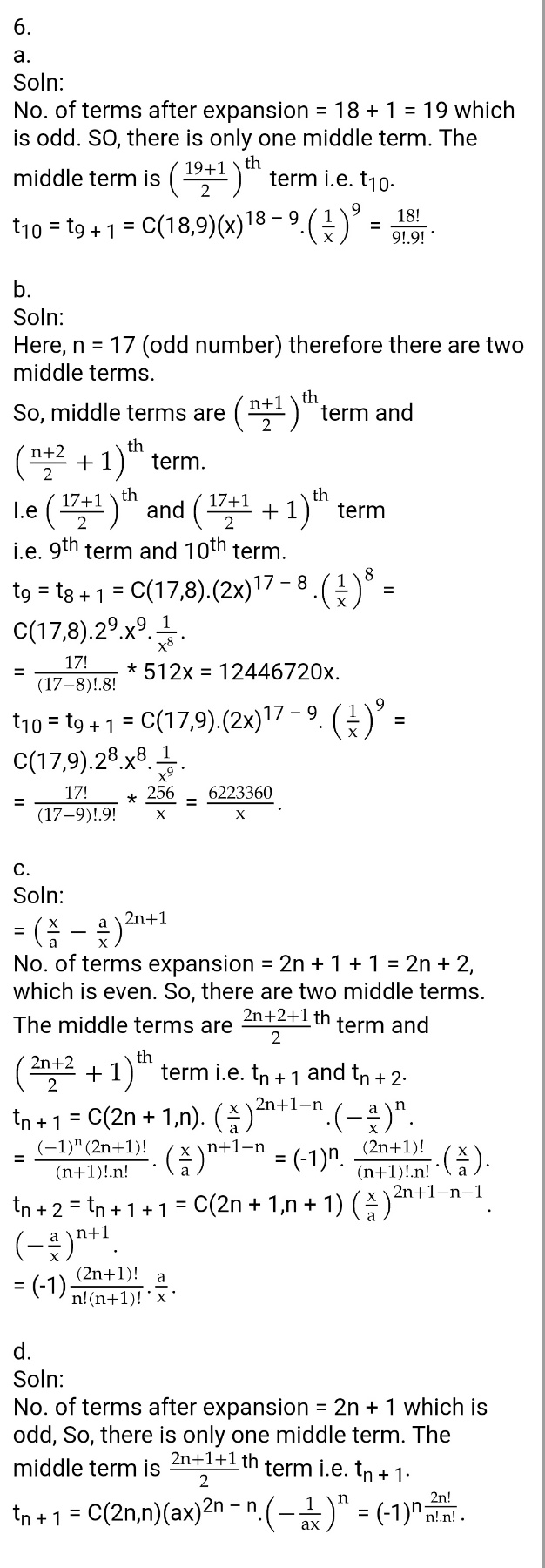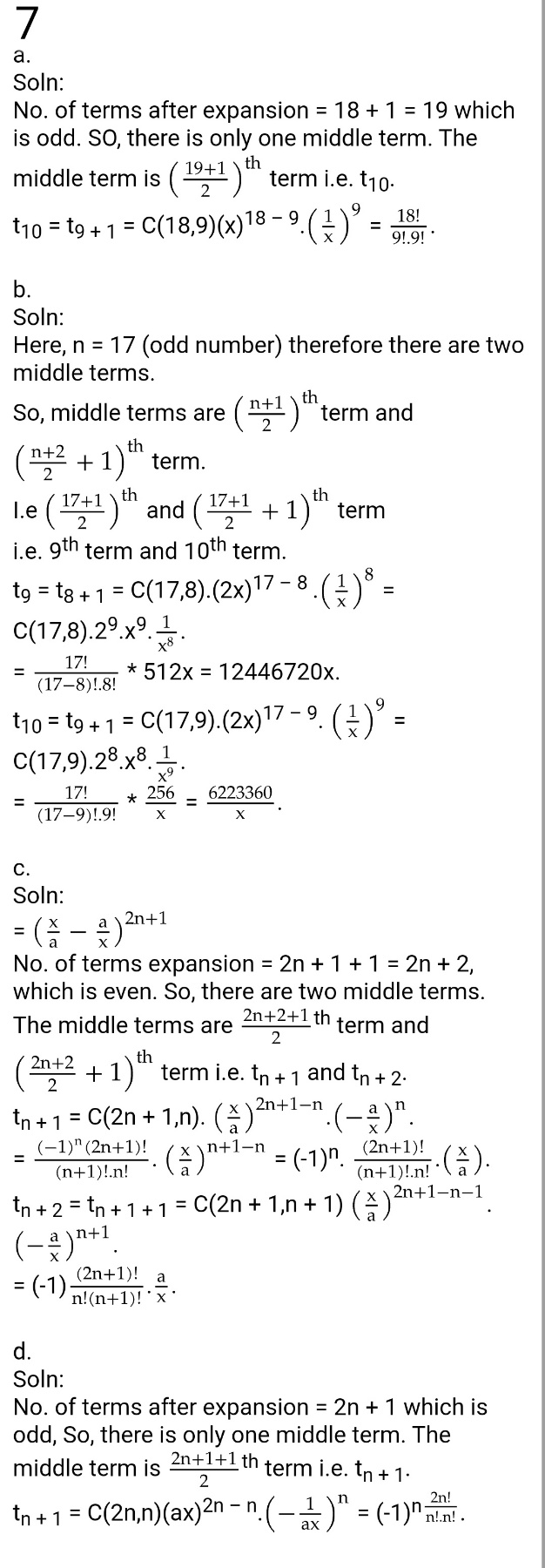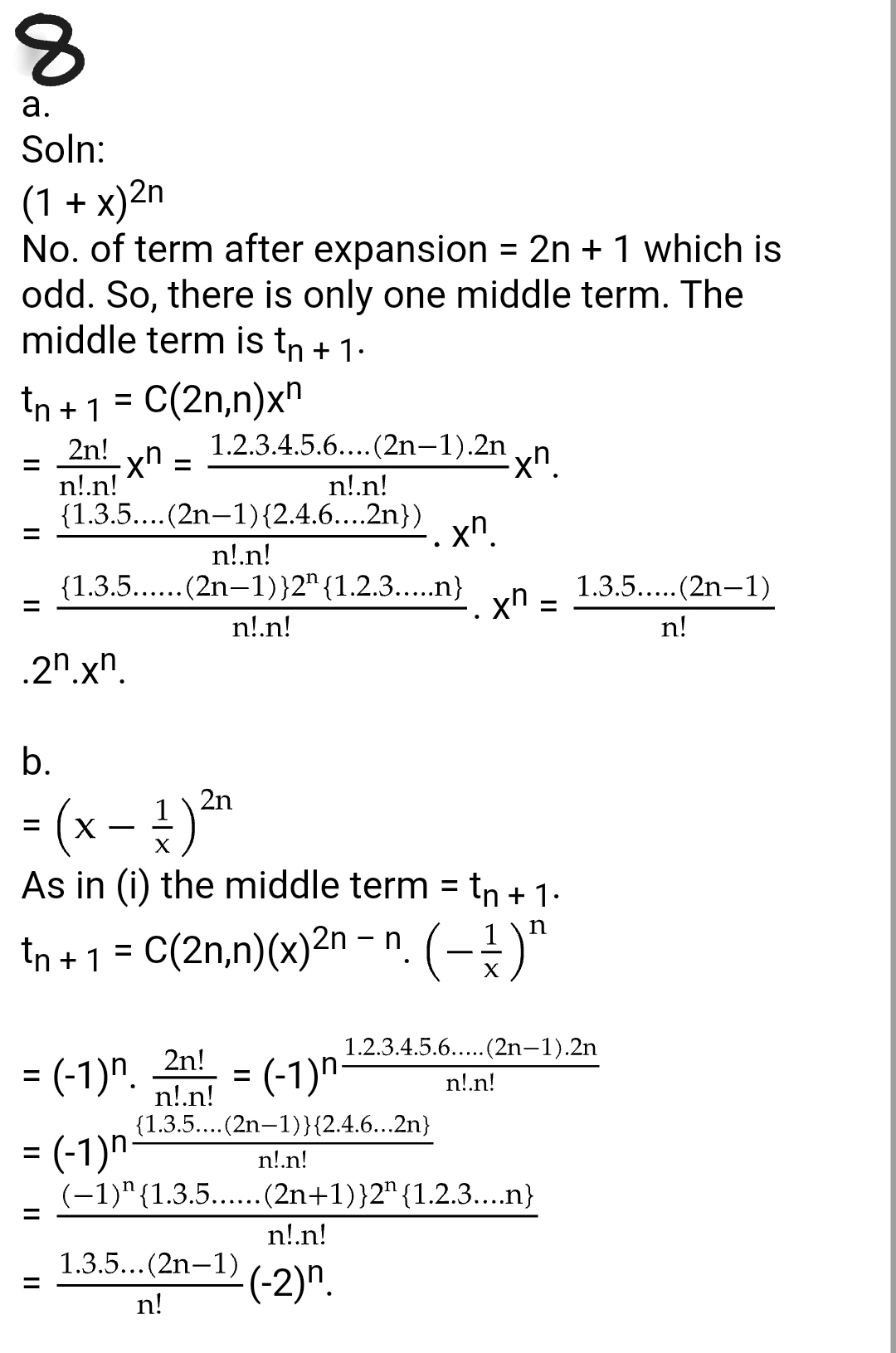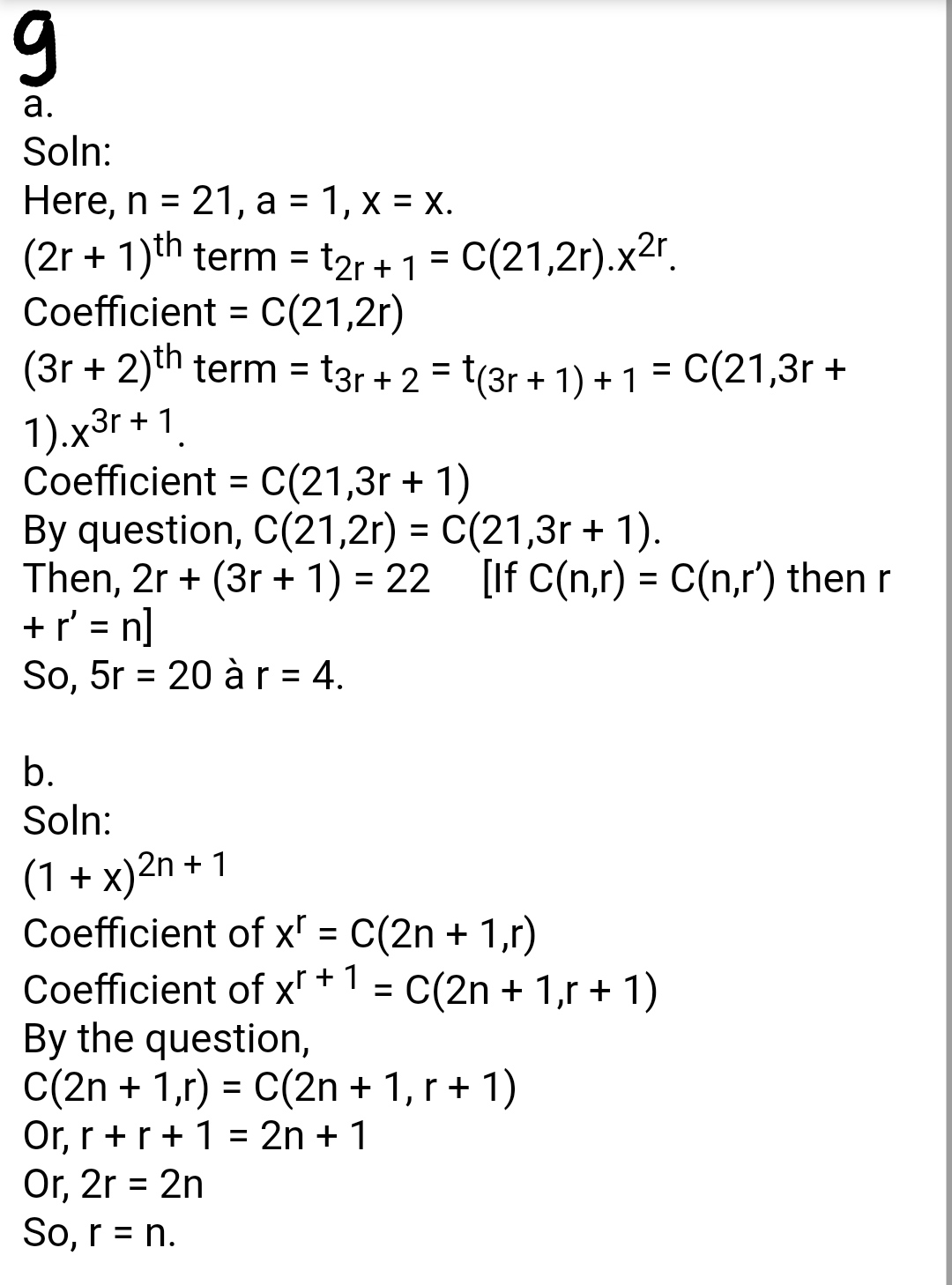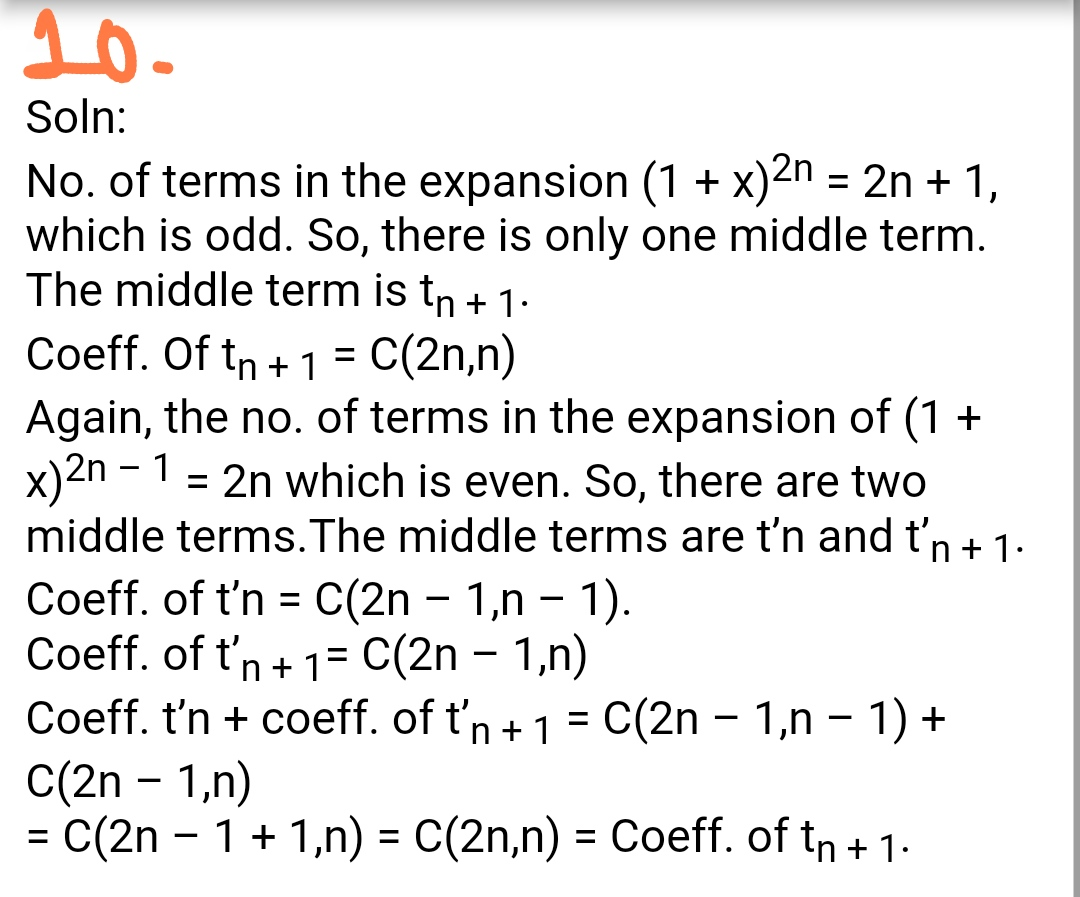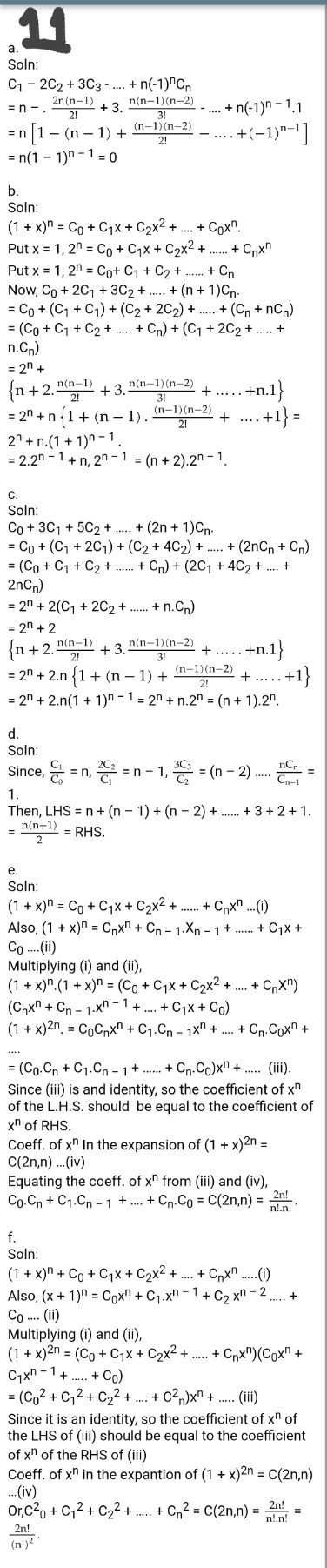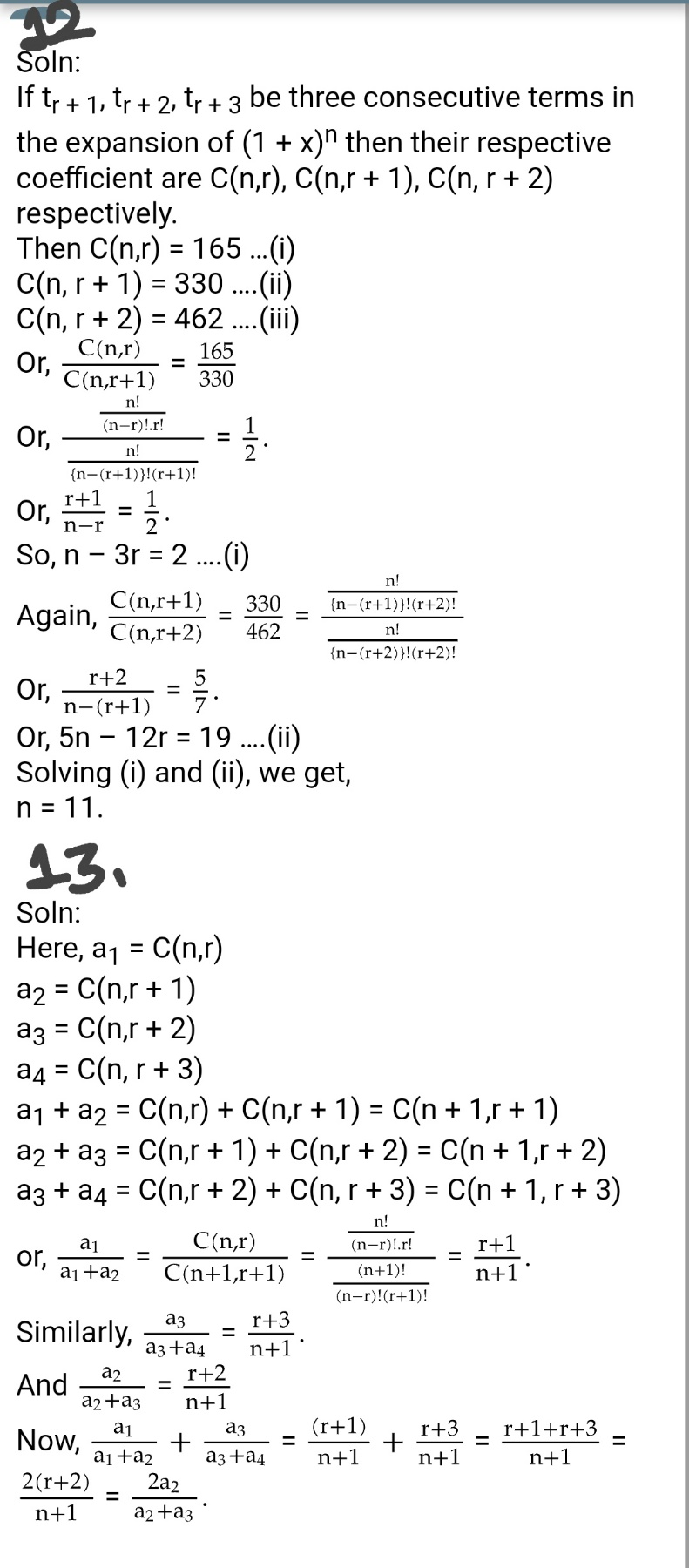Binomial Theorem - Exercise 2.2 : Class 12 Math

Binomial Theorem - Exercise 2.3 : Class 12 Math

Getting Info...